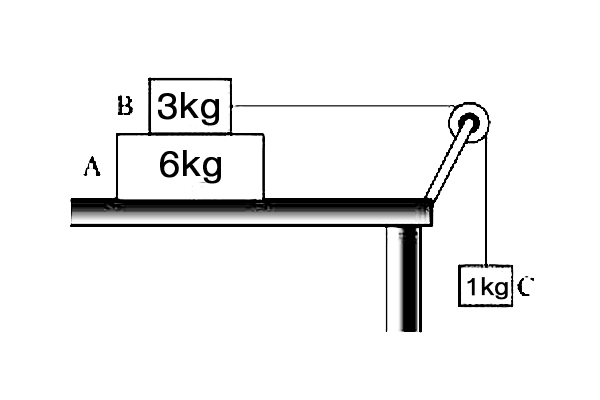# FrictionIn the diagram, object $A$ weighing $6 \text{ kg}$ is on a frictionless desk. Object $B$ weighing $3 \text{ kg}$ is sits atop $A,$ moving together with it due to friction. Object $C$ weighing $1 \text{ kg}$ is connected with object $B$ by a pulley.

Which of the following statements is correct?

$a)$ The magnitude of the force with which the string is pulling object $C$ is $9$ N.
$b)$ The direction of the friction force upon object $B$ is the same as the direction of motion of object $A.$
$c)$ The magnitude of the friction force upon object $B$ is $6$ N.

Gravitational acceleration is $g= 10 \text{ m/s}^2.$

×

Problem Loading...

Note Loading...

Set Loading...